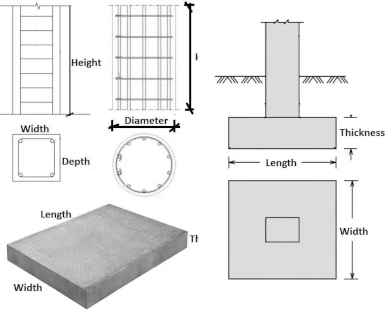# Estimating Concrete Quantities for Construction: Best Approaches

Accurate estimation of concrete quantities is a fundamental aspect of construction projects. Whether it's for foundations, slabs, columns, or other structural elements, precise calculations ensure that the right amount of concrete is ordered, minimizing waste and cost overruns. In this article, we explore the best approaches and formulas for estimating concrete quantities effectively.## 1. Understanding Concrete Volume

Before diving into calculations, it's crucial to understand how concrete volume is measured. Concrete volume is typically expressed in cubic yards or cubic meters. One cubic yard is equivalent to 27 cubic feet or approximately 0.7646 cubic meters. This unit of measurement forms the basis for most concrete quantity calculations.

## 2. Calculating Concrete for Slabs

Estimating concrete for slabs, such as floors or pavements, involves calculating the Volume of Concrete (V). The formula for a simple rectangular slab is:

V = Length × Width × Depth

Where Length and Width are the dimensions of the slab, and Depth is the thickness of the slab. For irregularly shaped slabs, the area may need to be divided into sections with different dimensions.

## 3. Estimating Concrete for Footings

Footings provide a stable base for structures, and their concrete volume needs to be accurately estimated. The formula for estimating the Concrete Volume of Footings (V) is:

V = Length × Width × Depth

Similar to slabs, the length, width, and depth dimensions are crucial. It's important to consider any additional volumes required for protruding parts of the footing, such as stepped footings.

## 4. Calculating Concrete for Columns

Columns are vertical load-bearing elements in a structure. The formula to calculate the Concrete Volume of a Column (V) is:

V = π × (Diameter / 2)^2 × Height

Where Diameter is the width of the column, and Height is its length. This formula assumes that the column is cylindrical in shape.

## 5. Factoring in Wastage

Concrete wastage is inevitable due to factors like spillage, over-excavation, and irregular shapes. To account for wastage, a common practice is to add a wastage factor to the estimated concrete quantity. This can range from 5% to 10% of the calculated volume, depending on the project's complexity and the contractor's experience.

## 6. Concrete Mix Design

Concrete mix design plays a crucial role in estimating quantities accurately. Different concrete mixes have varying water-cement ratios, affecting the final volume. It's essential to work with a specific mix design and adjust calculations accordingly. The concrete mix design provides details on the proportions of cement, water, aggregates, and admixtures, ensuring the desired strength and durability.

## 7. Estimating Concrete for Walls

Calculating concrete quantities for walls involves considering the wall's height, length, and thickness. The formula to estimate the Concrete Volume of Walls (V) is:

V = Height × Length × Thickness

For walls with openings, such as doors and windows, the wall can be divided into sections with different dimensions, and each section's volume can be calculated individually.

## 8. Concrete Pumping and Placing

When estimating concrete quantities, it's also essential to consider the logistics of concrete pumping and placing. The distance from the concrete plant to the construction site, the height and location of the pour, and the type of equipment used can impact the efficiency and accuracy of placing the concrete. This consideration helps prevent delays and ensures the right amount of concrete is available when needed.

## 9. Using Software and Tools

Estimating concrete quantities manually can be time-consuming and prone to errors. Construction professionals often rely on specialized software and tools to streamline the estimation process. These tools allow for quick input of dimensions, wastage factors, and mix designs, providing accurate and efficient results. Software solutions can also generate detailed reports and cost estimates for better project planning.

## 10. Consultation with Experts

While formulas and tools are valuable, consulting with experienced engineers, architects, and contractors is essential for precise concrete quantity estimation. Professionals bring real-world insights and project-specific considerations that can significantly impact the accuracy of calculations. Their expertise helps avoid potential pitfalls and ensures that all factors are taken into account.

## 11. Continuous Monitoring and Adjustments

Construction projects are dynamic, and changes can occur during different phases. Continuous monitoring of concrete quantities and regular adjustments based on site conditions and project modifications are essential. Flexibility in estimating allows for better resource allocation and cost management throughout the construction process.

## 12. Conclusion

Accurate estimation of concrete quantities is a crucial aspect of successful construction projects. By understanding the best approaches, utilizing appropriate formulas, considering wastage, focusing on concrete mix design, and leveraging technology and expert advice, construction professionals can ensure that the right amount of concrete is delivered to the site. This efficiency minimizes costs, reduces waste, and contributes to the overall quality of the project.

Remember, every construction project has its unique characteristics, so it's essential to adapt these approaches to suit the specific requirements and complexities of each job.